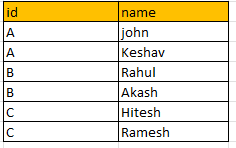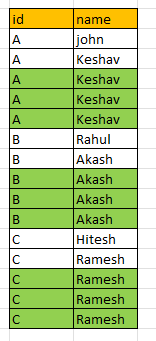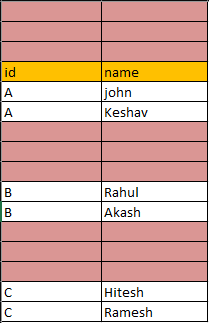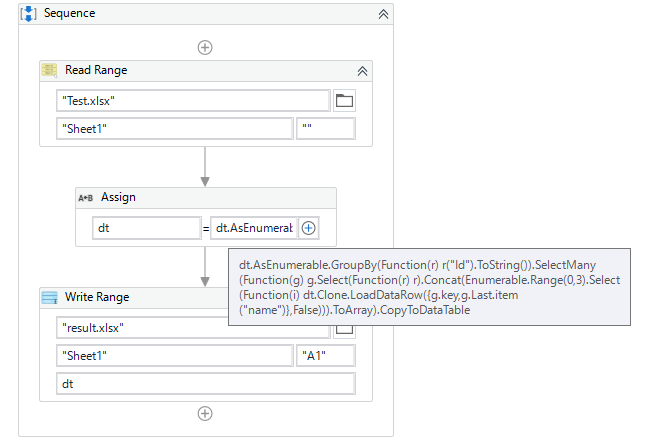# How to add 3rows to last of the every duplicate value and copy the data to it?

Input excel:Expected output:This is my workflow, but rows are getting added like this:Main.xaml (8.9 KB)
Test.xlsx (8.7 KB)

can anyone give me solution for this?

Hi,

Hope the following sample helps you.]

`````` dt = dt.AsEnumerable.GroupBy(Function(r) r("Id").ToString()).SelectMany(Function(g) g.Select(Function(r) r).Concat(Enumerable.Range(0,3).Select(Function(i) dt.Clone.LoadDataRow({g.key,g.Last.item("name")},False))).ToArray).CopyToDataTable
``````

Sample20220124-3.zip (8.8 KB)

Regards,

Thanks its working
Can you explain this expression in simple words?

Hi,

It is easier to understand if we break it down into some expressions.

``````dt = dt.AsEnumerable.GroupBy(Function(r) r("Id").ToString()).SelectMany(Function(g) g.Select(Function(r) r).Concat(Enumerable.Range(0,3).Select(Function(i) dt.Clone.LoadDataRow({g.key,g.Last.item("name")},False))).ToArray).CopyToDataTable
``````

`dt.AsEnumerable.GroupBy(Function(r) r("Id").ToString())` makes group by content of Id column.

`SelectMany(Function(g)` translate multiple arrays to single array.

`g.Select(Function(r) r).Concat` means returning rows in the group and concatenate the followign array.

`Enumerable.Range(0,3).Select(Function(i) dt.Clone.LoadDataRow({g.key,g.Last.item("name")},False))).ToArray)` returns 3 rows which has key of the group and last item of name column of the group.

As a result, this returns the above datatable.

Regards,

is there any different way to implement this same process?

Hi,

is there any different way to implement this same process?

Hope the following sample helps you.

Sample20220124-4.zip (9.4 KB)

Regards,

1 Like

This topic was automatically closed 3 days after the last reply. New replies are no longer allowed.